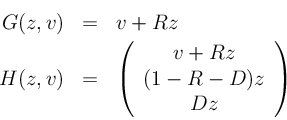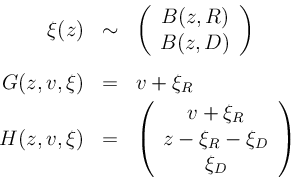### Theory-based implementation

• Implementation of Class A as state space model:
• define variables
• z ≡ x, v ≡ i, w ≡ (c, t, d)'
• define G and H
•• implementation trivial
• Implementation of Class B as probabilistic state space model:
• add two-dimensional random vector ξ = (ξR, ξD)
• define G, H and ξ
•• implementation uses Binomial block from above